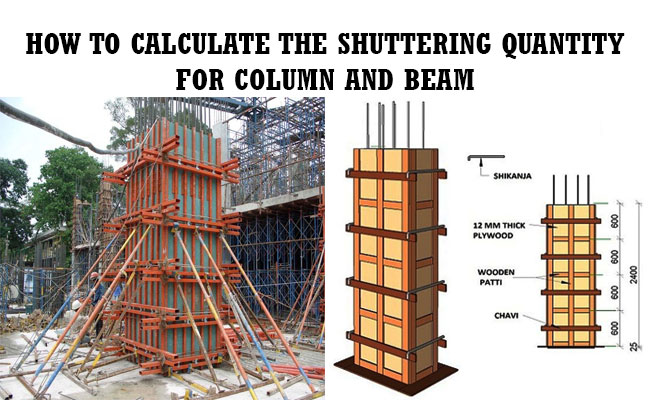### How to calculate the shuttering quantity for column and beamThere are different types building estimation methods like long wall-short wall method, center line method, partly center line & short wall method.

In this construction article, you will learn how to measure the quantity of shuttering in square meter for column and beam at the time doing RCC work and building work.

Initially, useful guidelines are given for measuring the shuttering quantity for column. For this purpose, the dimension of the is required.

The dimension of the column is taken as follow :-

L x W x H = 300 (0.3m) mm x 200 mm (0.2m) x 3 m

For rectangular column, the following formula is used :-

Width of column x Height of column x similar face

Size of column = 200 x 300

Suppose, the sides of the column are taken as A and B.

The area of side A will be calculated as follow :-

Width x Length x Number of face = 0.3 x 3 x 2 (for both opposite sides) =1.8 square meter

The above formula will also be applied to determine the area of side B.

So, side B = 0.2 x 3 x 2 = 1.2 square meter

So, total shuttering area for square column = 1.8 + 1.2 = 3 square meter

So, for doing building work, 3 square meter shuttering will be required.

For square column, area of the all the sides will be same. So, in this case, the calculation will be done for only one side i.e 0.2 x 3 x 4.

Quantity of shuttering for rectangular beam

The calculation is done with the following formula :-

Size of beam is taken as 200 mm x 400 mm

Width of beam x Length of beam x Similar face

Side A = Depth x Length x Similar Face = 0.4 x 4 x 2 = 3.2 square meter

Bottom = Width x Length = 0.22 x 4 = 0.88 square meter

Length is provided from inside column to inside column.

So, total shuttering area = 3.20 + 0.88 = 4.08 square meter